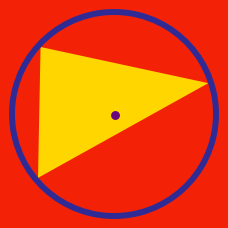Geometry

# Triangle Centers: Level 4 Challenges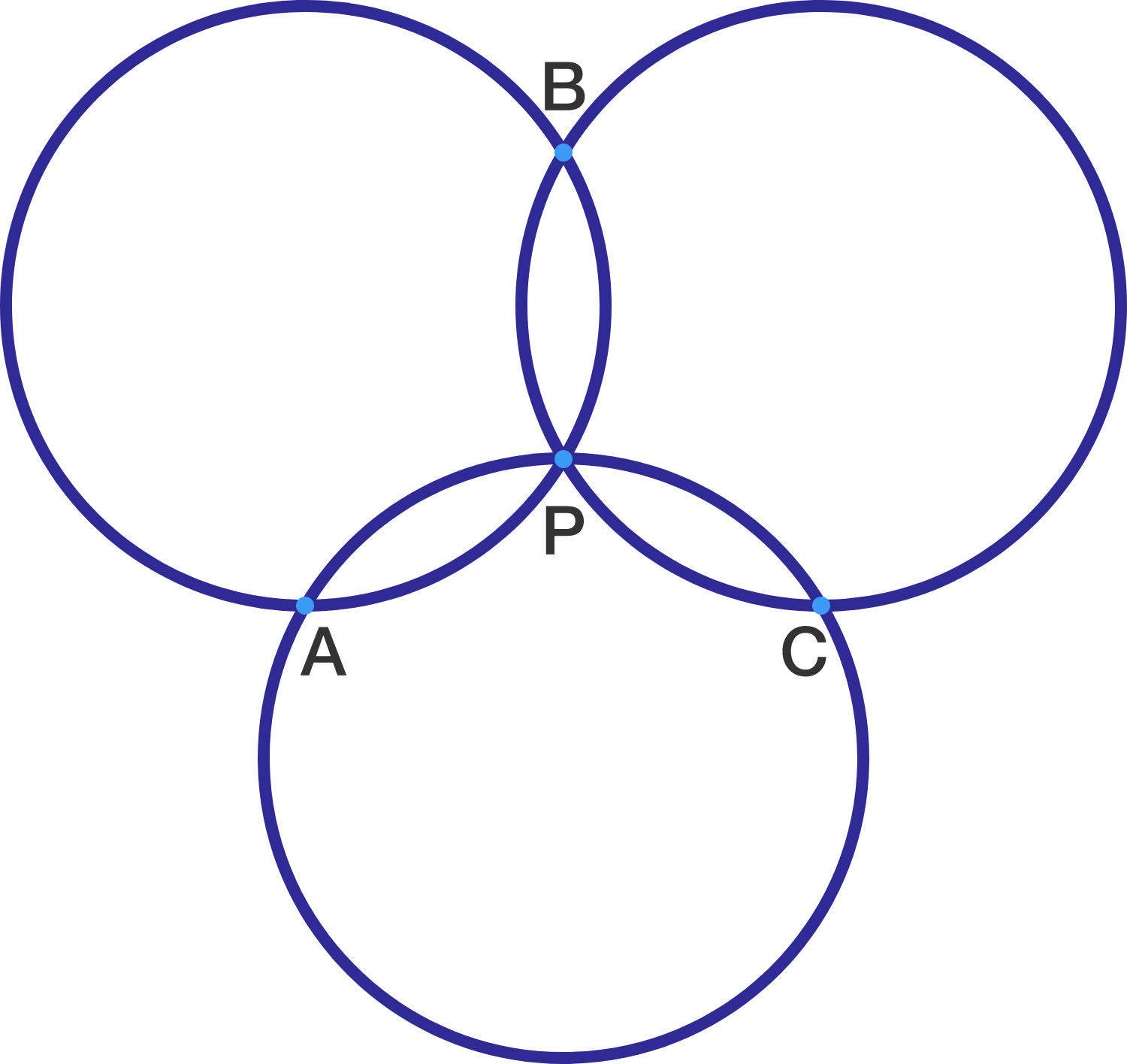Three circles of equal radii all intersect at a single point $P$. Let the other intersections be $A$, $B$ and $C$. Which of the following must be true?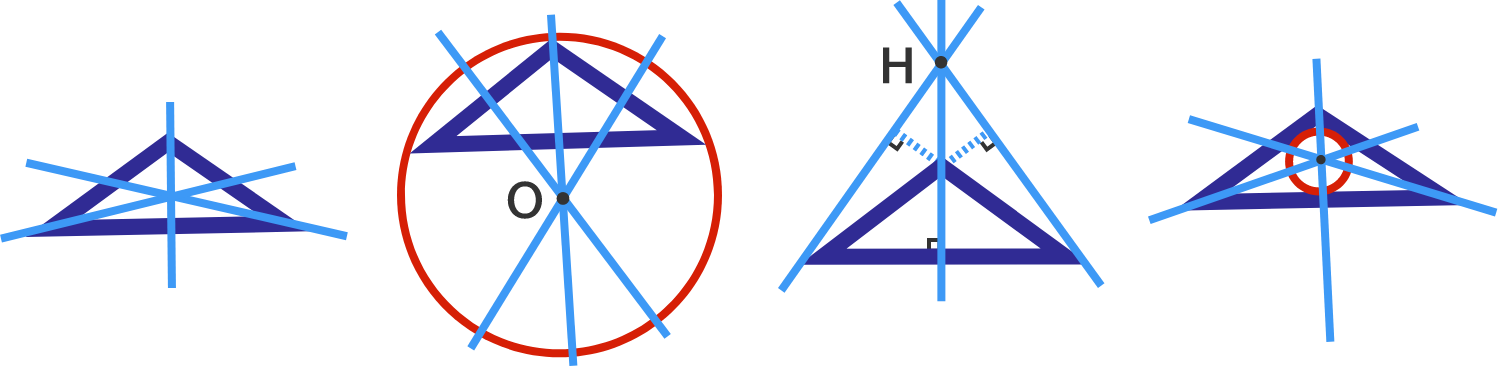If the vertices of a triangle have rational coordinates, then the coordinates of which of the following are necessarily rational?

(A) Centroid
(B) Circumcenter
(C) Orthocenter
(D) Incenter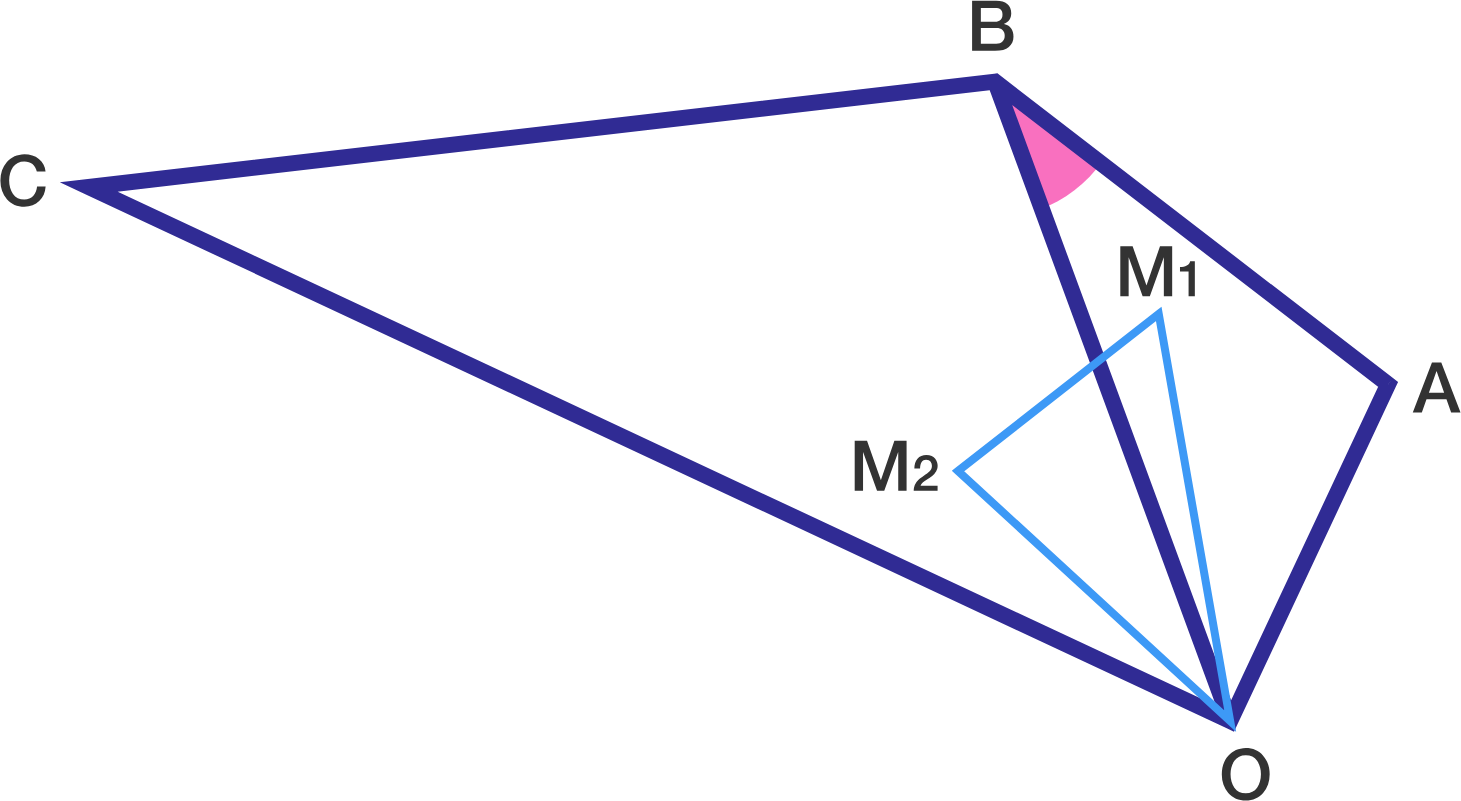Triangle $AOB$ and triangle $BOC$ are directly similar (they have the same orientation). Let the centroids of these two triangles be $M_1$ and $M_2$, respectively.

If triangle $M_1OM_2$ is right, and $M_1M_2=AB$, then the sum of all possible values of $\cos^2(\angle ABO)$ can be expressed as $\dfrac{p}{q}$ for positive coprime integers $p,q$.

Find $p+q$.

##### Note: We do not know which angle of $\triangle M_1 O M_2$ is the right angle.

Let $ABC$ be a triangle in which $AB=AC$. Suppose the orthocentre of the triangle lies on the incircle.

Find $\dfrac{AB}{BC}$.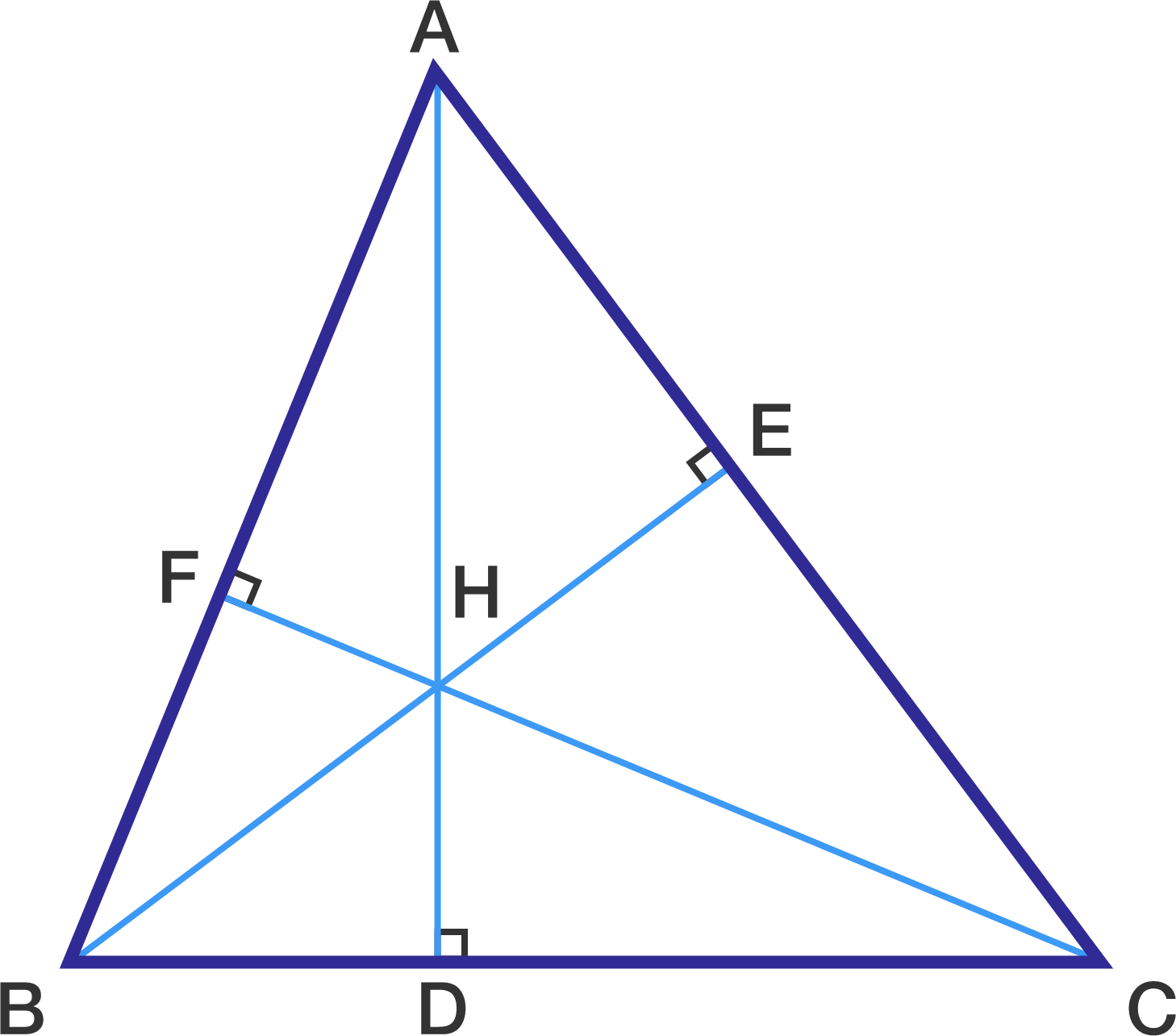Consider $\Delta ABC$ such that $AB = 13, BC = 14, AC = 15$

Let $AD \perp BC, BE \perp AC, CF \perp AB$ and let $H$ be its orthocenter

Let $R(ABC)$ denote circumradius of $\Delta ABC$

Let $r_0$ be inradius and $r_1, r_2, r_3$ be the exradii of $\Delta ABC$

Then find the value of

$R(ABC) + R(HAB) + R(HBC) + R(HAC) + \sum_{i=0}^3 r_i$

×